Courses

# Chapter 11 Turbulent Flow - Fluid Mechanics, Mechanical Engineering Mechanical Engineering Notes | EduRev

## Mechanical Engineering : Chapter 11 Turbulent Flow - Fluid Mechanics, Mechanical Engineering Mechanical Engineering Notes | EduRev

The document Chapter 11 Turbulent Flow - Fluid Mechanics, Mechanical Engineering Mechanical Engineering Notes | EduRev is a part of the Mechanical Engineering Course Mechanical Engineering SSC JE (Technical).
All you need of Mechanical Engineering at this link: Mechanical Engineering

TURBULENT FLOW

• Velocity distribution is relatively uniform and velocity profile is much flatter than the corresponding laminar flow parabola for the same mean velocity, as shown below :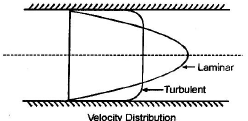• Shear stress in turbulent flow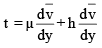where, µ = dynamic coefficient of viscosity (fluid characteristic) h = eddy viscosity coefficient (flow characteristic) 

• eddy viscosity come into picture due to turbulence effect

• Hydro-Dynamically Smooth And Rough Pipes 
• If the average height of irregularities (k) is greater than the thickness of laminar sublayer (d'), then the boundary is called hydrodynamically Rough. 
• If the average height of irregularities (k) is less than the thickness of laminar sublayer (d'), then the boundary is called hydrodynamically smooth. 
• On the basis of NIKURADSE's EXPERIMENT the boundary is classified as :

Hydrodynamically smooth : k/d < 0.25'

Boundary in transition :6.0 < k/d < 0.25

Hydrodynamically Rough : k/d > 6.0

• R/K is known as specific roughness. where ‘k’ is average height of roughness and‘R’ is radius of the pipe. 

• Velocity Distribution For Turbulent Flow in Pipes

(a) Prandtl’s universal velocity distribution equation :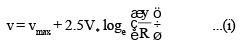where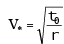= shear or friction velocity..

y = distance from pipe wall R = radius of pipe. 

• The above equation is valid for both smooth and rough pipe boundaries.
(b) Karman - Prandtl Velocity distribution equation :
(i) Hydro Dynamically Smooth pipe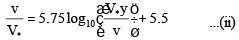(ii) Hydro Dynamically Rough pipe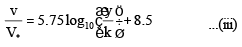where
V* = shear velocity y = distance from pipe wall k = average height of roughness v = kinematic viscosity.
(c) Velocity distribution in terms of mean velocity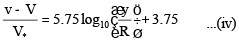The above equation is for both rough and smooth pipes. 

• Friction Factor
(a) Friction factor ‘f ’ for laminar flow :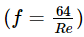where Re = Reynolds number

(b) Friction factor ‘f ’ for transition flow :

There exists no specific relationship between f and Re for transition flow in pipes.

(c) Friction factor (f) for turbulent flow in smooth pipes :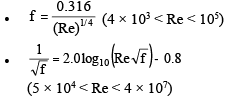(d) Friction factor (f) for turbulent flow in rough pipes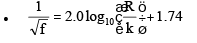This equation shows that for rough pipes friction factor depends only on R/K (Relative smoothness) and not on Reynolds number (Re)

Offer running on EduRev: Apply code STAYHOME200 to get INR 200 off on our premium plan EduRev Infinity!

83 docs|53 tests

,

,

,

,

,

,

,

,

,

,

,

,

,

,

,

,

,

,

,

,

,

,

,

,

;